# Pure submodule

(diff) ← Older revision | Latest revision (diff) | Newer revision → (diff)

in the sense of Cohn

A submoduleof a right-modulesuch that for any left-modulethe natural homomorphism of Abelian groupsis injective. This is equivalent to the following condition: If the system of equationshas a solution in, then it has a solution in(cf. Flat module). Any direct summand is a pure submodule. All submodules of a right-module are pure if and only ifis a regular ring (in the sense of von Neumann).

In the case of Abelian groups (that is,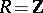), the following assertions are equivalent: 1)is a pure (or serving) subgroup of(cf. Pure subgroup); 2)for every natural number; 3)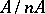is a direct summand of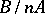for every natural number; 4) if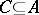and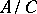is a finitely-generated group, thenis a direct summand of; 5) every residue class in the quotient group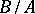contains an element of the same order as the residue class; and 6) ifand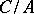is finitely generated, thenis a direct summand of. If property 2) is required to hold only for prime numbers, thenis called a weakly-pure subgroup.

The axiomatic approach to the notion of purity is based on the consideration of a class of monomorphism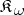subject to the following conditions (here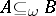means thatis a submodule ofand that the natural imbedding belongs to): P0') ifis a direct summand of, then; P1') ifand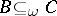, then; P2') ifand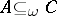, then; P3') ifand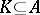, then; and P4') if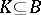,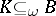and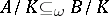, then. Taking the classinstead of the class of all monomorphisms leads to relative homological algebra. For example, a moduleis called-injective ifimplies that any homomorphism fromintocan be extended to a homomorphism frominto(cf. Injective module). A pure injective Abelian group is called algebraically compact. The following conditions on an Abelian groupare equivalent:)is algebraically compact;)splits as a direct summand of any group that contains it as a pure subgroup;)is a direct summand of a group that admits a compact topology; and) a system of equations overis solvable if every finite subsystem of it is solvable.

How to Cite This Entry:
Pure submodule. Encyclopedia of Mathematics. URL: http://encyclopediaofmath.org/index.php?title=Pure_submodule&oldid=18846
This article was adapted from an original article by L.A. Skornyakov (originator), which appeared in Encyclopedia of Mathematics - ISBN 1402006098. See original article